# RS Aggarwal Solutions for Class 9 Chapter 3: Factorisation of Polynomials Exercise 3A

## RS Aggarwal Solutions for Class 9 Maths Exercise 3A PDF

This chapter is basically a continuation of the previous chapter with concepts of higher importance to be understood by the students. The students can acquire a better grip on the subject by regular practice and getting their doubts clarified by using the RS Aggarwal Solutions. The problems solved with explanations in simple language by the faculties help students to perform well in the exams. Exercise 3A of the third chapter mainly contains problems based on the factorisation methods and ways of grouping polynomials by using factorisation. RS Aggarwal Solutions for Class 9 Maths Chapter 3 Factorisation of Polynomials Exercise 3A are provided here.

## RS Aggarwal Solutions for Class 9 Chapter 3: Factorisation of Polynomials Exercise 3A Download PDF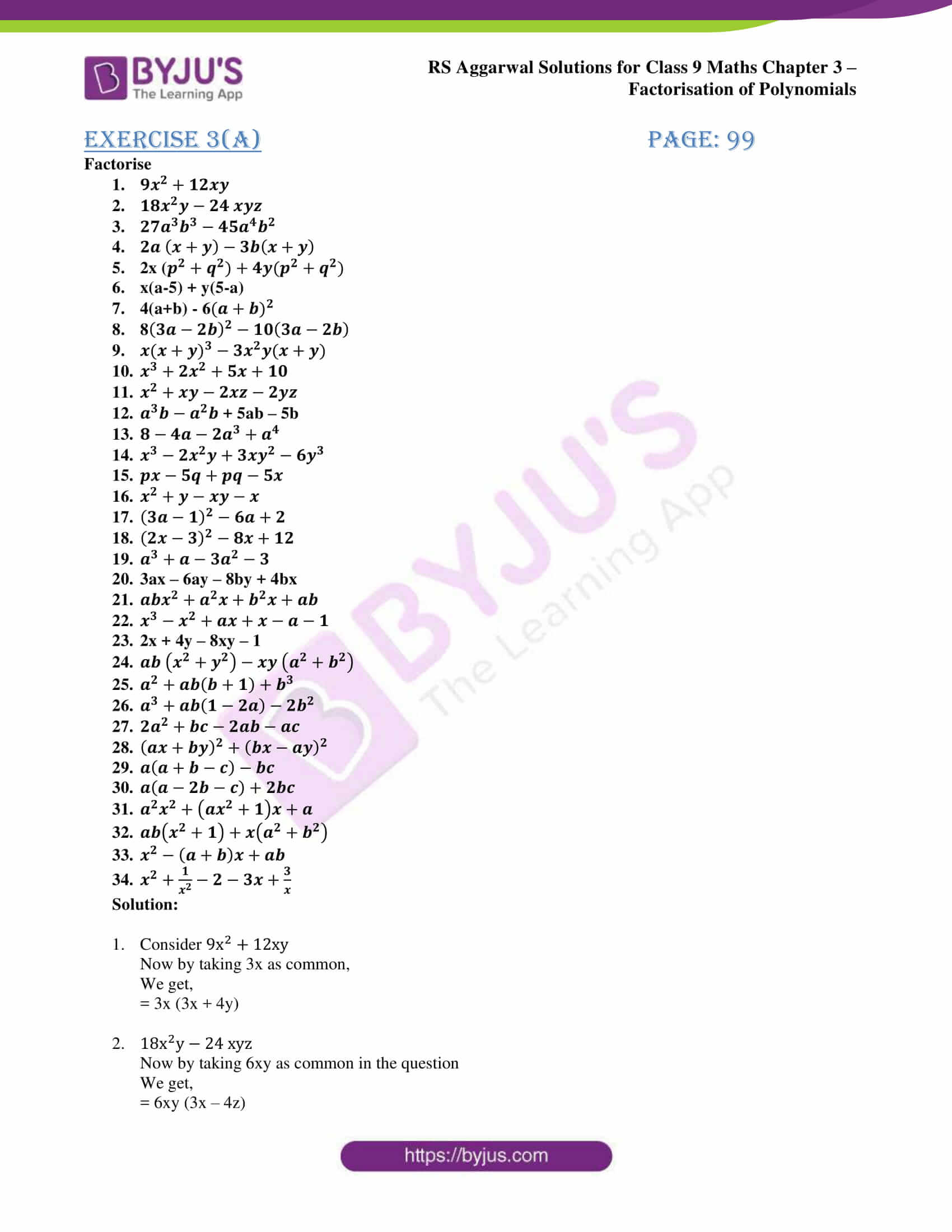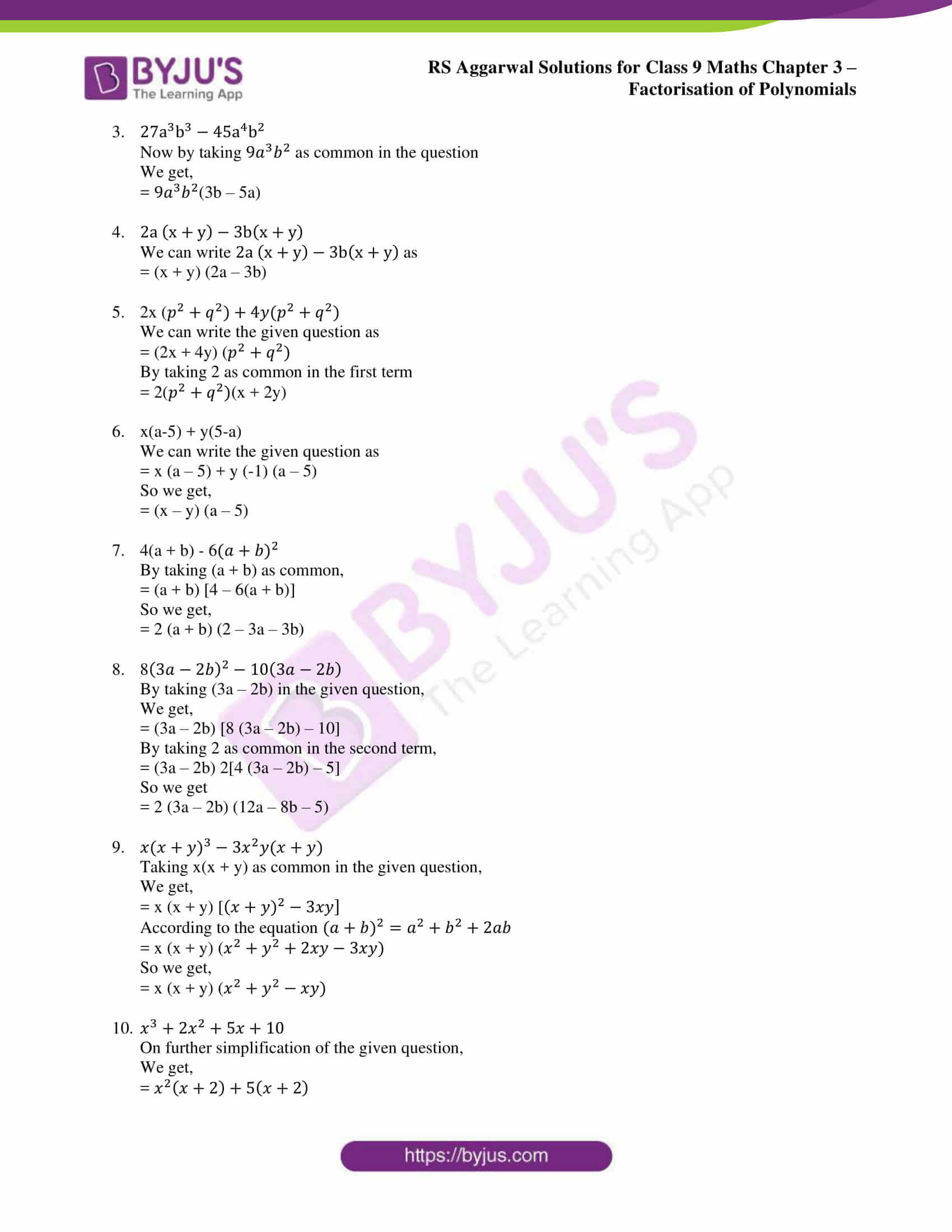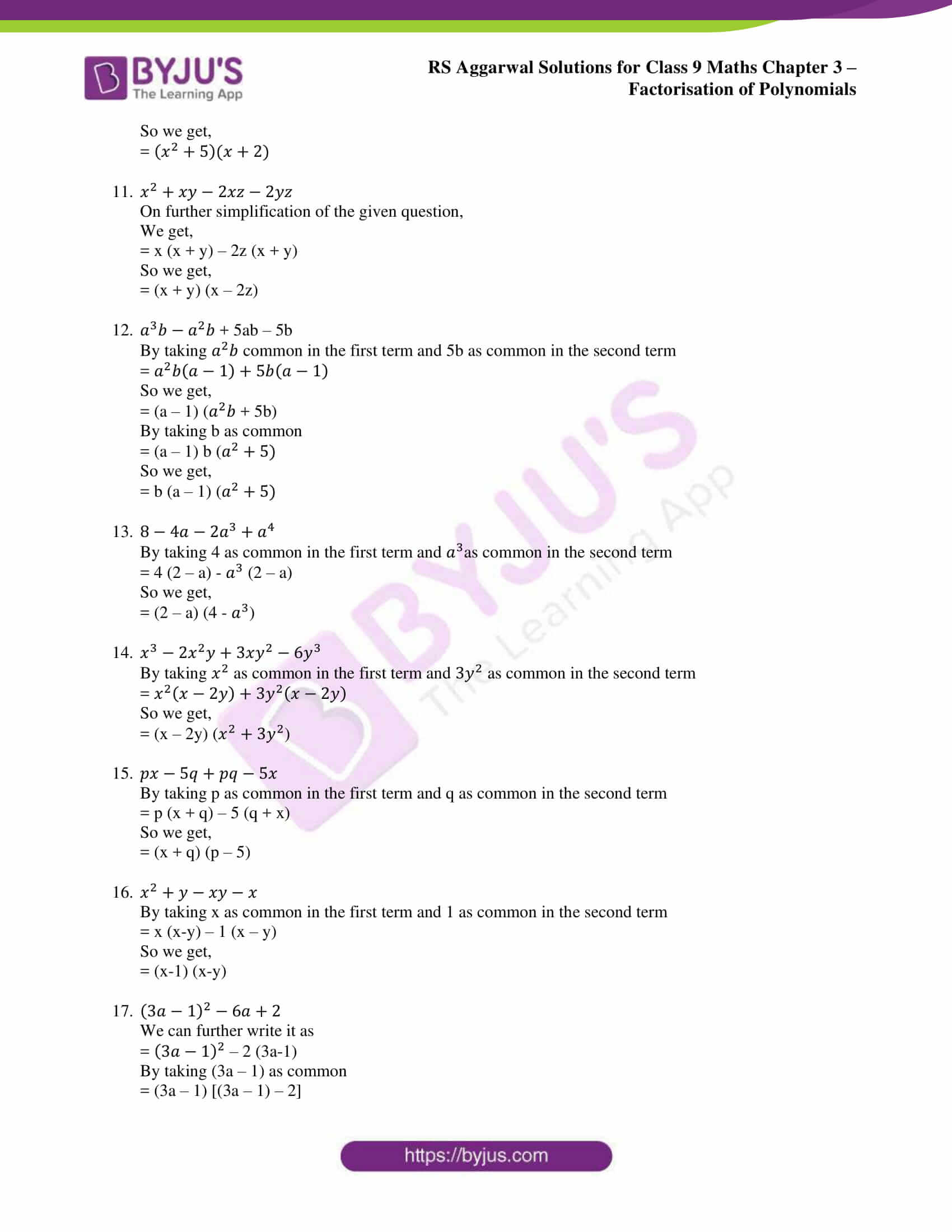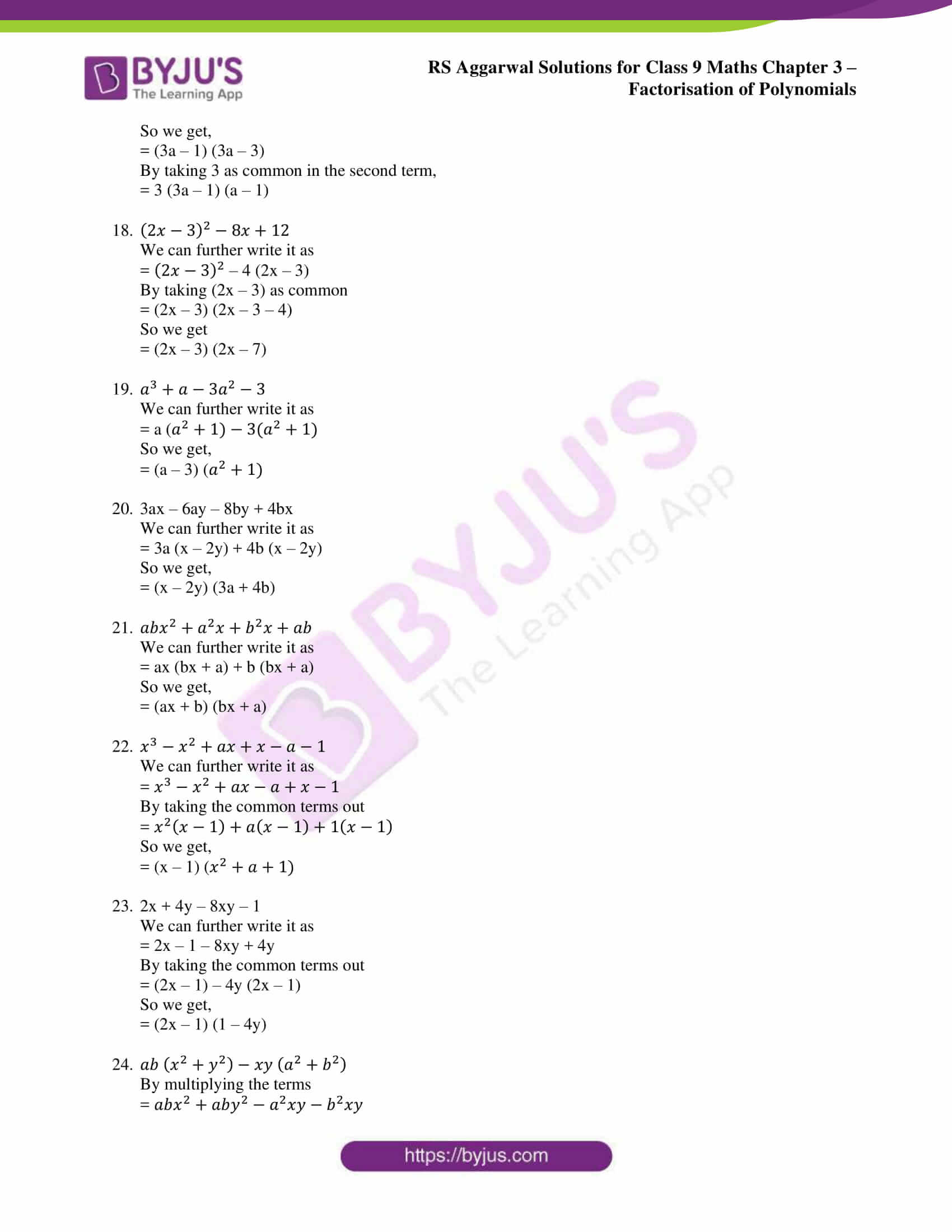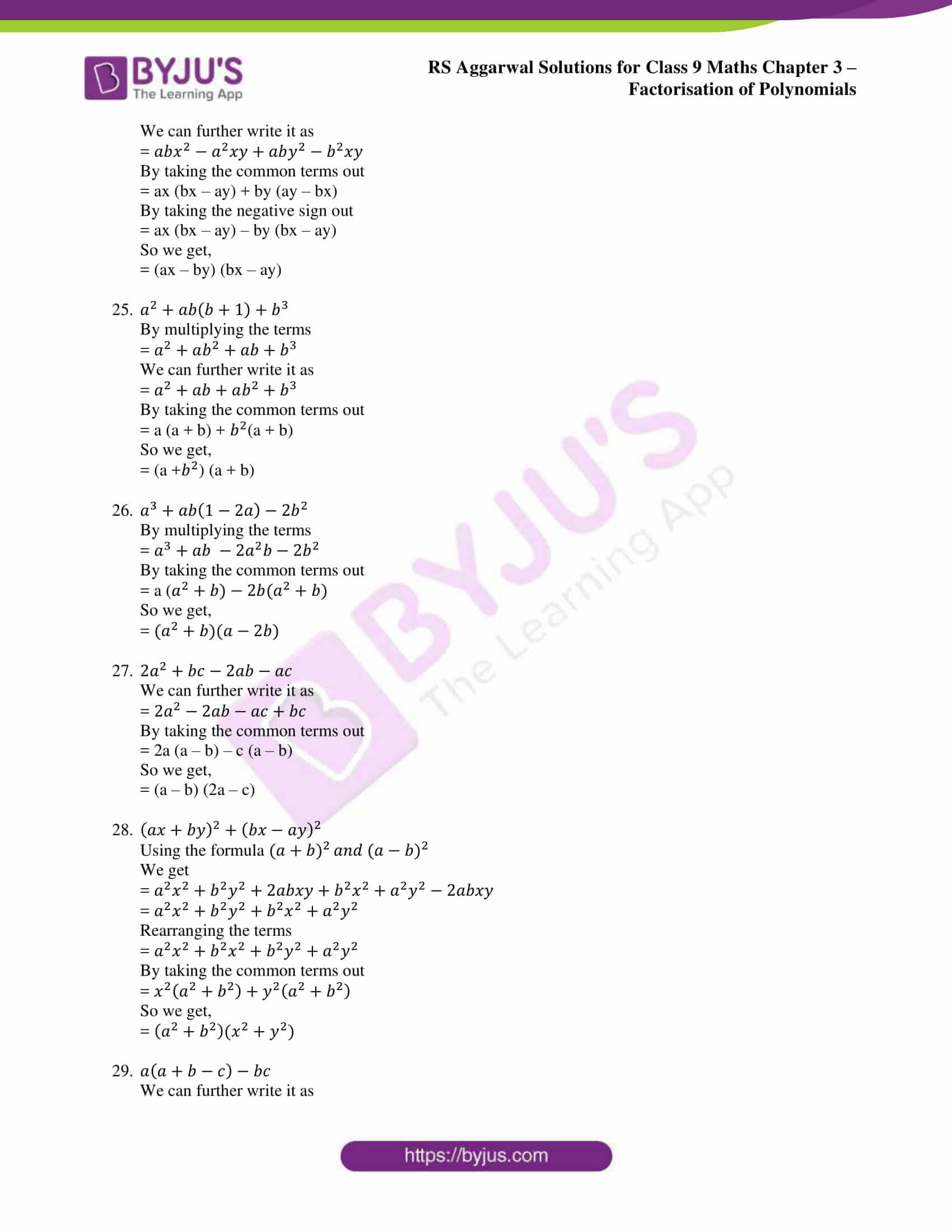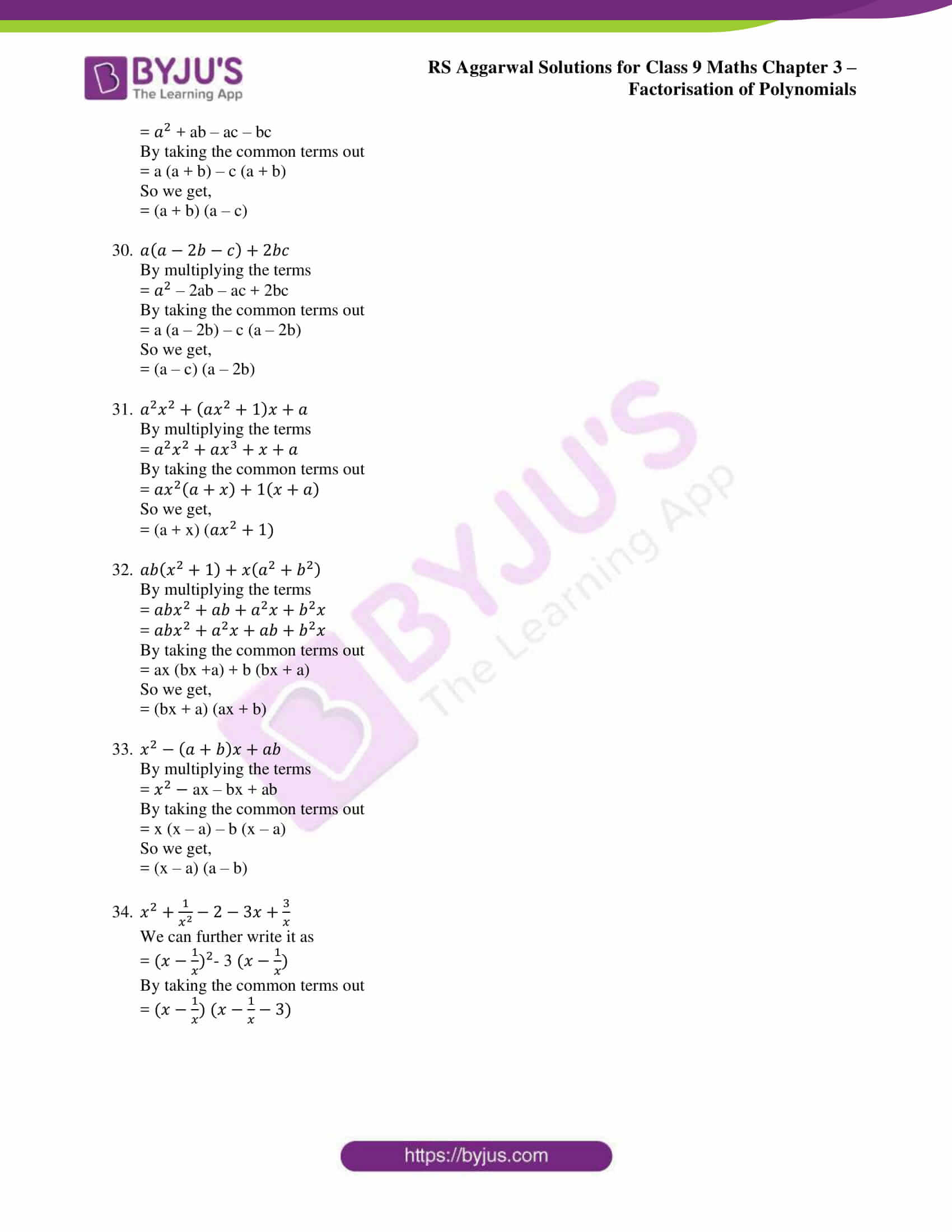### Access other exercise solutions of class 9 Maths Chapter 3: Factorisation of Polynomials

Exercise 3B Solutions 40 Questions

Exercise 3C Solutions 66 Questions

Exercise 3D Solutions 7 Questions

Exercise 3E Solutions 10 Questions

Exercise 3F Solutions 38 Questions

Exercise 3G Solutions 25 Questions

### RS Aggarwal Solutions Class 9 Maths Chapter 3 – Factorisation of Polynomials Exercise 3A

RS Aggarwal Solutions Class 9 Maths Chapter 3 Factorisation of Polynomials Exercise 3A contains a set of problems solved based on various concepts like Factorisation, Methods of Factorisation and Factorisation by grouping.

### Key features of RS Aggarwal Solutions for Class 9 Maths Chapter 3: Factorisation of Polynomials Exercise 3A

• Exam preparation is made easier for the students by using the RS Aggarwal Solutions for Class 9.
• Solutions are simple and solved in various methods, thereby ensuring students can use the method which is easiest for them while practising.
• PDF format of solutions for each exercise is present in the BYJU’S website which can be downloaded by the students and referred for studying.
• The solutions are prepared from the exam point of view of students based on RS Aggarwal textbook of the current CBSE syllabus.